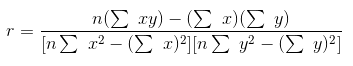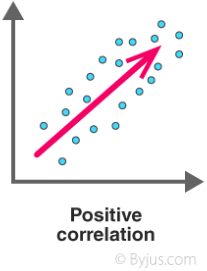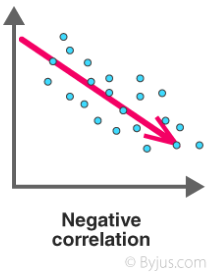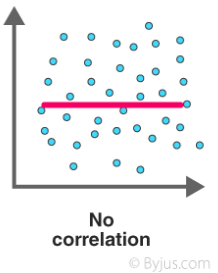# Correlation Coefficient

Correlation Coefficient is a statistical concept, which helps in establishing a relation between predicted and actual values obtained in a statistical experiment. The calculated value of the correlation coefficient explains the exactness between the predicted and actual values.

Correlation Coefficient value always lies between -1 to +1. If correlation coefficient value is positive, then there is a similar and identical relation between the two variables. Else it indicates the dissimilarity between the two variables.

The covariance of two variables divided by the product of their standard deviations gives Pearson’s correlation coefficient. It is usually represented by ρ (rho).

ρ (X,Y) = cov (X,Y) / σX.σY.

Here cov is the covariance. σX is the standard deviation of X and σY is the standard deviation of Y. The given equation for correlation coefficient can be expressed in terms of means and expectations.

$$\begin{array}{l}\rho (X,Y)= E\frac{(X-\mu _{x})(Y-\mu _{y})}{\sigma x.\sigma y}\end{array}$$

μx and μy are mean of x and mean of y respectively. E is the expectation.

## Assumptions of Karl Pearson’s Correlation Coefficient

The assumptions and requirements for calculating Pearson’s correlation coefficient are as follows:

1. The data set which is to be correlated should approximate to the normal distribution. If the data is normally distributed, then the data points tend to lie closer to the mean.

2. The word homoscedastic is a greek originated meaning ‘able to disperse’. Homoscedasticity means ‘equal variances’. For all the values of the independent variable, the error term is the same. Suppose the error term is smaller for a certain set of values of independent variable and larger for another set of values, then homoscedasticity is violated. It can be checked visually through a scatter plot. The data is said to be homoscedastic if the points lie equally on both sides of the line of best fit.

3. When the data follows a linear relationship, it is said to be linearity. If the data points are in the form of a straight line on the scatter plot, then the data satisfies the condition of linearity.

4. The variables which can take any value in an interval are continuous variables. The data set must contain continuous variables to compute the Pearson correlation coefficient. If one of the data sets is ordinal, then Spearman’s rank correlation is an appropriate measure.

5. The data points must be in pairs which are termed as paired observations. There exists a dependent variable for every observation of the independent variable.

6. There must be no outliers in the data. If the outliers are present, then they can skew the correlation coefficient and make it inappropriate. A point is considered to be an outlier if it is beyond +3.29 or -3.29 standard deviations away. They can be easily determined visually from a scatter plot.

## Pearson Correlation Coefficient Formula

The linear correlation coefficient defines the degree of relation between two variables and is denoted by “r”. It is also called as Cross correlation coefficient as it predicts the relation between two quantities. Now let us proceed to a statistical way of calculating the correlation coefficient.

 If x & y are the two variables of discussion, then the correlation coefficient can be calculated using the formulaHere,

n = Number of values or elements

$$\begin{array}{l}\sum\end{array}$$
x = Sum of 1st values list

$$\begin{array}{l}\sum\end{array}$$
y = Sum of 2nd values list

$$\begin{array}{l}\sum\end{array}$$
xy = Sum of the product of 1st and 2nd values

$$\begin{array}{l}\sum\end{array}$$
x2 = Sum of squares of 1st values

$$\begin{array}{l}\sum\end{array}$$
y2 = Sum of squares of 2nd values

## How to find the Correlation Coefficient

Correlation is used almost everywhere in statistics. Correction illustrates the relationship between two or more variables. It is expressed in the form of a number that is known as correlation coefficient. There are mainly two types of correlations:

• Positive Correlation
• Negative Correlation
 Positive Correlation The value of one variable increases linearly with increase in another variable. This indicates a similar relation between both the variables. So its correlation coefficient would be positive or 1 in this case.Negative Correlation When there is a decrease in values of one variable with increase in values of other variable. In that case, correlation coefficient would be negative.Zero Correlation or No Correlation There is one more situation when there is no specific relation between two variables.## Correlation Coefficient Properties

Correlation coefficient is all about establishing relationships between two variables. Some properties of correlation coefficient are as follows:

1) Correlation coefficient remains in the same measurement as in which the two variables are.

2) The sign which correlations of coefficient have will always be the same as the variance.

3) The numerical value of correlation of coefficient will be in between -1 to + 1. It is known as real number value.

4) The negative value of coefficient suggests that the correlation is strong and negative. And if ‘r’ goes on approaching toward -1 then it means that the relationship is going towards the negative side.

When ‘r’ approaches to the side of + 1 then it means the relationship is strong and positive. By this we can say that if +1 is the result of the correlation then the relationship is in a positive state.

5) The weak correlation is signaled when the coefficient of correlation approaches to zero. When ‘r’ is near about zero then we can deduce that the relationship is weak.

6) Correlation coefficient can be very dicey because we cannot say that the participants are truthful or not.

The coefficient of correlation is not affected when we interchange the two variables.

7) Coefficient of correlation is a pure number without effect of any units on it. It also not get affected when we add the same number to all the values of one variable. We can multiply all the variables by the same positive number. It does not affect the correlation coefficient. As we discussed, ‘r ‘is not affected by any unit because ‘r’ is a scale invariant.

8) We use correlation for measuring the association but that does not mean we are talking about causation. By this, we simply mean that when we are correlating the two variables then it might be the possibility that the third variable may be influencing them.

## Examples on Correlation Coefficient

Example 1: Calculate the Correlation coefficient of given data:

 x 50 51 52 53 54 y 3.1 3.2 3.3 3.4 3.5

Solution:

Here n = 5

 x 50 51 52 53 54 y 3.1 3.2 3.3 3.4 3.5 xy 155 163.2 171.6 180.2 189 x2 2500 2601 2704 2809 2916 y2 9.61 10.24 10.89 11.56 12.25

sum x = 260

sum y = 16.5

sum xy = 859

sum x2 = 13530

sum y2 = 54.55

By substituting all the values in formula, we get r = 1. This shows a positive correlation coefficient.

Example 2: Calculate the Correlation coefficient of given data:

 x 12 15 18 21 27 y 2 4 6 8 12

Solution:

Here n = 5

 x 12 15 18 21 27 y 2 4 6 8 12 xy 24 60 94 168 324 x2 144 225 324 441 729 y2 4 16 36 64 144

sum x = 93

sum y = 32

sum xy = 670

sum x2 = 1863

sum y2 = 264

Now, putting all the values in below formulaWe have, r = 0.84

Statistics for IIT JEE

Mean and Variance of random variables

## Cramer’s V Correlation

Cramer’s V Correlation is identical to the Pearson Correlation coefficient. Pearson Correlation coefficient is used to find the correlation between variables whereas Cramer’s V is used in the calculation of correlation in tables with more than 2 x 2 columns and rows. It varies between 0 and 1. 0 indicates less association between the variables whereas 1 indicates a very strong association.

Cramer’s V

.25 or higher – very strong relationship
.15 to .25 – strong relationship
.11 to .15 – moderate relationship
.06 to .10 – weak relationship
.01 to .05 – No or negligible relationship

### Other types of correlation are as follows:

1] Concordance Correlation coefficient
It measures the bivariate pairs of observations comparative to a “gold standard” measurement.

2] Intraclass Correlation
It measures the reliability of the data that are collected as groups.

3] Kendall’s Tau
It is a non-parametric measure of relationships between the columns of ranked data.

4] Moran’s I
It measures the overall spatial autocorrelation of the data set.

5] Partial Correlation
It measures the strength of a relationship between two variables while controlling for the effect of one or more other variables.

6] Phi Coefficient
It measures the association between two binary variables.

7] Point Biserial Correlation: It is a special case of Pearson’s correlation coefficient. It measures the relationship between two variables:
a] One continuous variable.
b] One naturally binary variable.

8] Spearman Rank Correlation
It is the nonparametric version of the Pearson correlation coefficient.

9] Zero-Order Correlation
It indicates nothing has been controlled for or “partialed out” in an experiment.

### What do you mean by correlation coefficient?

Correlation coefficient is a statistical concept used to measure how strong a relationship is between two variables.

### Give the formula for the Pearson’s correlation coefficient.

Pearson’s correlation coefficient is given by ρ (X,Y) = cov (X,Y)/σX.σY.

### What is the range of the correlation coefficient?

The value of the correlation coefficient lies between -1 to +1.

### What do you mean by zero correlation?

A zero correlation denotes that the correlation statistic does not indicate a relationship between the two variables.

Test your Knowledge on correlation coefficient